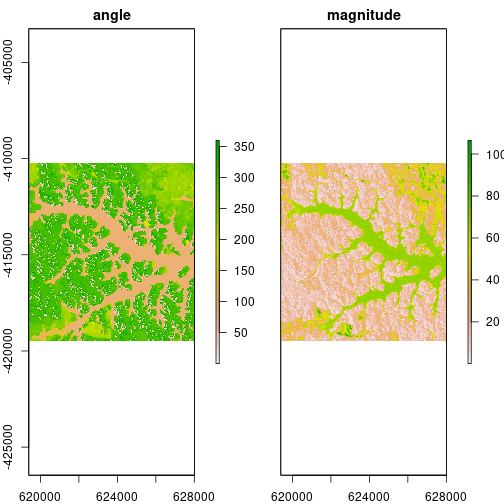rasterCVA { RStoolbox} R Documentation

### Change Vector Analysis

R: Change Vector Analysis

### Description

Calculates angle and magnitude of change vectors. Dimensionality is limited to two bands per image.

### Usage

```rasterCVA(x, y, tmf = NULL, nct = NULL, ...)
```

### Arguments

 `x` RasterBrick or RasterStack with two layers. This will be the reference/origin for the change calculations. Both rasters (y and y) need to correspond to each other, i.e. same resolution, extent and origin. `y` RasterBrick or RasterStack with two layers. Both rasters (y and y) need to correspond to each other, i.e. same resolution, extent and origin. `tmf` Numeric. Threshold median factor (optional). Used to calculate a threshold magnitude for which pixels are considered stable, i.e. no change. Calculated as `tmf * mean(magnitude[magnitude > 0])`. `nct` Numeric. No-change threshold (optional). Alternative to `tmf`. Sets an absolute threshold. Change magnitudes below `nct` are considered stable and set to NA. `...` further arguments passed to writeRaster

### Details

Change Vector Analysis (CVA) is used to identify spectral changes between two identical scenes which were acquired at different times. CVA is limited to two bands per image. For each pixel it calculates the change vector in the two-dimensional spectral space. For example for a given pixel in image A and B for the red and nir band the change vector is calculated for the coordinate pairs: (red_A | nir_A) and (red_B | nir_B).

The coordinate system is defined by the order of the input bands: the first band defines the x-axis and the second band the y-axis, respectively. Angles are returned *in degree* beginning with 0 degrees pointing 'north', i.e. the y-axis, i.e. the second band.

### Value

Returns a RasterBrick with two layers: change vector angle and change vector magnitude

### Examples

``````library(raster)
## Create example data
data(lsat)
pca <- rasterPCA(lsat)\$map

## Do change vector analysis
cva <- rasterCVA(pca[[1:2]], pca[[3:4]])
cva
``````
``````#> class      : RasterBrick
#> dimensions : 310, 287, 88970, 2  (nrow, ncol, ncell, nlayers)
#> resolution : 30, 30  (x, y)
#> extent     : 619395, 628005, -419505, -410205  (xmin, xmax, ymin, ymax)
#> crs        : +proj=utm +zone=22 +datum=WGS84 +units=m +no_defs +ellps=WGS84 +towgs84=0,0,0
#> source     : memory
#> names      :       angle,   magnitude
#> min values : 0.005423571, 0.100938665
#> max values :    359.9993,    106.5001
``````
``````plot(cva)
``````[Package RStoolbox version 0.3.0 Index]